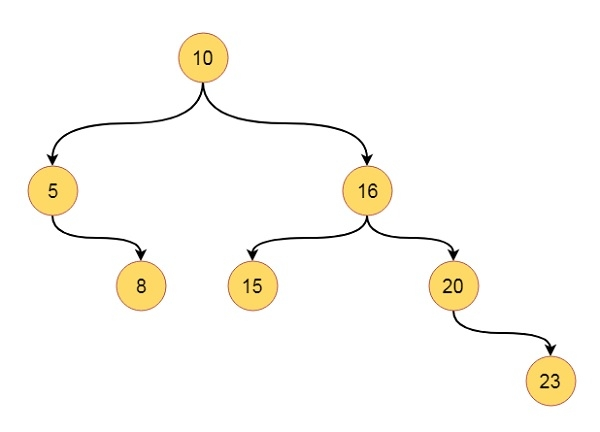# Level Order Tree Traversal in Data Structures

In this section we will see the level-order traversal technique for binary search tree.

Suppose we have one tree like this −The traversal sequence will be like: 10, 5, 16, 8, 15, 20, 23

## Algorithm

levelOrderTraverse(root):
Begin
define queue que to store nodes
insert root into the que.
while que is not empty, do
item := item present at front position of queue
print the value of item
if left of the item is not null, then
insert left of item into que
end if
if right of the item is not null, then
insert right of item into que
end if
delete front element from que
done
End

## Example

Live Demo

#include<iostream>
#include<queue>
using namespace std;
class node{
public:
int h_left, h_right, bf, value;
node *left, *right;
};
class tree{
private:
node *get_node(int key);
public:
node *root;
tree(){
root = NULL; //set root as NULL at the beginning
}
void levelorder_traversal(node *r);
node *insert_node(node *root, int key);
};
node *tree::get_node(int key){
node *new_node;
new_node = new node; //create a new node dynamically
new_node->h_left = 0; new_node->h_right = 0;
new_node->bf = 0;
new_node->value = key; //store the value from given key
new_node->left = NULL; new_node->right = NULL;
return new_node;
}
void tree::levelorder_traversal(node *root){
queue <node*> que;
node *item;
que.push(root); //insert the root at first
while(!que.empty()){
item = que.front(); //get the element from the front end
cout << item->value << " ";
if(item->left != NULL) //When left child is present, insert into queue
que.push(item->left);
if(item->right != NULL) //When right child is present, insert into queue
que.push(item->right);
que.pop(); //remove the item from queue
}
}
node *tree::insert_node(node *root, int key){
if(root == NULL){
return (get_node(key)); //when tree is empty, create a node as root
}
if(key < root->value){ //when key is smaller than root value, go to the left
root->left = insert_node(root->left, key);
} else if(key > root->value) { //when key is greater than root value, go to the right
root->right = insert_node(root->right, key);
}
return root; //when key is already present, do not insert it again
}
main(){
node *root;
tree my_tree;
//Insert some keys into the tree.
my_tree.root = my_tree.insert_node(my_tree.root, 10);
my_tree.root = my_tree.insert_node(my_tree.root, 5);
my_tree.root = my_tree.insert_node(my_tree.root, 16);
my_tree.root = my_tree.insert_node(my_tree.root, 20);
my_tree.root = my_tree.insert_node(my_tree.root, 15);
my_tree.root = my_tree.insert_node(my_tree.root, 8);
my_tree.root = my_tree.insert_node(my_tree.root, 23);
cout << "Level-Order Traversal: ";
my_tree.levelorder_traversal(my_tree.root);
}

## Output

Level-Order Traversal: 10 5 16 8 15 20 23

Updated on: 27-Aug-2019

240 Views